# How the Table Calculations Use Building Formulas in Tableau

Rating: 5

6059

## Building Formulas Using Table Calculations

In contrast to Calculated Values, Quick Table calculations use the data in your Visualization to create a formula. Before you can use Quick Table calculations you must first create a worksheet that includes Visualization. Using Superstore again, below diagram displays a time series of monthly sales on top. The bottom half employs a Quick Table calculation to derive the running total of sales as the year progresses.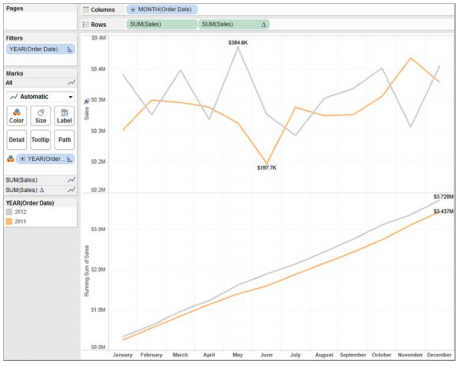Time series using a running sum diagram

The steps required to build the charts in below diagram are:

1. Add month to the column shelf.
2. Add sales to the row shelf.
3. Filter order date for the year(s) 2011 and 2012.
4. Add order date to the color marks button.
5. Turn on labeling for min/max values.
Mindmajix Tableau Training helps you master in building interactive tableau dashboards and learn data visualization tools. Start Learning!

The data from the sales time-series chart will serve as the data source for a quick table calculation that will be used to create the chart in the bottom half of below diagram. That chart displays the running sum of sales for each month within the displayed years. The steps required to add that portion of the view are:

1. Ctrl drag the sales pill on the row shelf to create a duplicate chart.
2. Right-click on the second sales pill.
3. Select quick table calculation-running total.
4. Turn on field labels for the line ends and un-check label start of line.

The below diagram shows how right-clicking on the duplicate sales pill exposes the quick table calculation menu.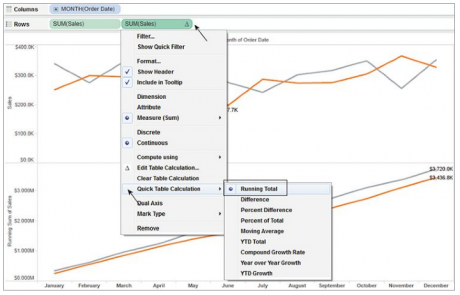Diagram of creating the quick table calculation

Checkout Tableau Interview Questions

Selecting running total, generates the table calculation that results in the running total time series chart. The label number format was also formatted to display the results in thousands in the top chart and millions in the lower chart. The total time required to build this chart was less than 60 seconds.
Editing table calculations to suit your purpose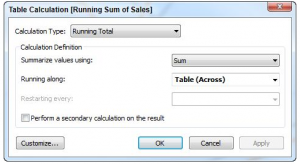The table calculation editing menu diagram

You can also seen in below diagram that there are many other quick table calculation option available. There is also a menu option called edit table calculation in fact, the four rows in the menu below continuous are all used to customize table calculations.

Understanding how table calculations works take a little time for playing with the options and looking at the results. Take a close look at the edit table calculation menu option displayed in below diagram
Table Calculations require selections of the following options:

• Calculation type— as seen in below diagram
• Aggregation method— sum, average, median, (these will change depending on the content of your source)
• Running Along— defines the direction that the calculation travels (Table Across, Table Down, etc)

The Restarting Every option is grayed-out in below diagram because there are no discrete time or other dimension panes dividing the Time Series. Modifying the Time Series to show time as discrete quarters and months creates quarterly partitions as seen in the below diagram.

The bottom Time Series showing the Running Sum of Sales is still using Table Across to calculate the total. Right-clicking on the table calculation (denoted by a small triangle on the right side of the pill) and selecting the Edit Table Calculation Menu exposes the Running Along control. Figure below shows the Table Calculation editing menu for Running Along and includes more options. Adding the partition for quarter creates quarterly panes that can be used in the Table Calculation.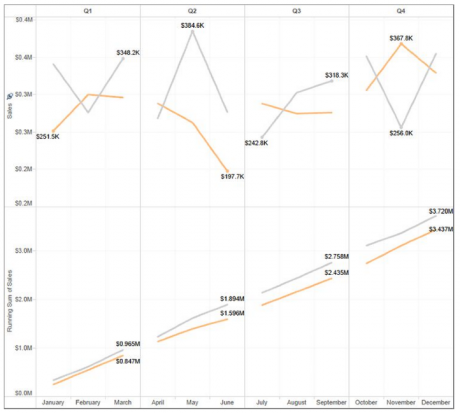Using discrete quarter and month diagram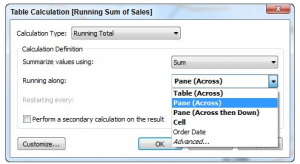Changing table calculation scope diagram

Changing the scope of the calculation to pane across causes the running sum calculation to reset every quarter (pane). Figure below reflects the revised scope in the lower pane. As you see, the running totals restart at the beginning of each quarter.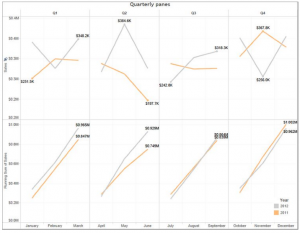Running sum set to pane across Diagram

### Understanding table calculation behavior

Learning exactly how table calculations behave in different visualizations takes a little time. The best way to learn is to build a crosstab report, then start playing with different options to see the results. Tableau’s online manual provides many different examples. Figure below shows Percent of Total table calculations using all of the different standard Running Along scope options.

Checkout Tableau Server Tutorials

Notice that in this example the example for the Table scope returns exactly the same result as the Table Down Then Across scope. Also, the Cell scope is calculating the mark value of itself, resulting in 100 percent in every cell. Depending on the structure of your view it is not uncommon for different scope options to return the same values. In general, adding more dimensions to your view will increase the number of available options provided by Table Calculations. Experiment with different Visualization styles and Table Calculations. With practice you’ll be able to anticipate how they behave in different situations.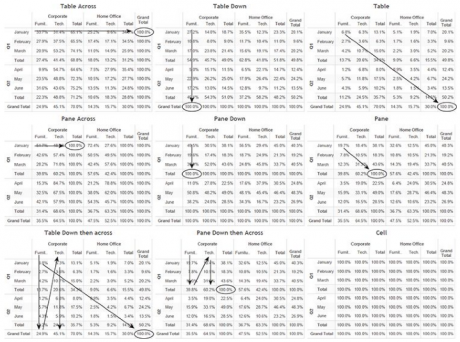Diagram of comparison of different scope options

### Customizing table calculations

Quick table calculations don’t normally result in calculated values appearing on the measures shelf so what if you want to use the result returned by a quick table calculation on another worksheet? Is that possible? Table calculation functions enable you do to this.

(Related Page: What Are The Common Use Cases For Tabcmd In Tableau?)

Refer to below diagram, the quick table calculation was used generating a running sum of sales chart at the bottom. To create a calculated value using that table calculation as the source requires just a couple of steps. Right-clicking on the table calculation pill used in that chart exposes the table calculation dialog box. Below diagram shows how to convert the quick table calculation into a calculated value that is reusable in another worksheet.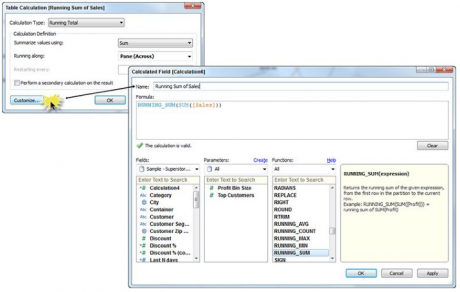Diagram of Creating a calculated value from a quick table calculation

Clicking the customize button causes the calculated value editing window to open with the formula already completed. Notice the formula employs a table calculation function running sum and the sum aggregation function together to create a new calculated value. The name field is used to type in the name that will appear on the measures shelf  when the OK button is clicked. Now this table calculation can be reused in a new worksheet. Below diagram shows one possible way that it could be deployed.

In this worksheet, all four years are displayed using the customized running sum of sales measure. Notice the new measure highlighted in green on the measures shelf. The row shelf displays the new measure as a table calculation.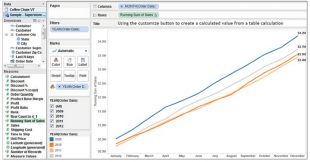Diagram of using the customized table calculation in another worksheet

### Secondary table calculations

Secondary table calculations allow you to pass the result of an initial table calculation to a second table calculation. The next example will use some data from the United States Census. The file includes state population figures. In the next example you’ll see how a Secondary Table Calculation can be used to enhance the population analysis.

The source spreadsheet is a small list that includes the state name along with the population tally. An initial view of the data shows the population information in a bar chart, sorted by descending population. You can see the result in below diagram.

Each state is placed in order from the largest to smallest in descending order. The population values are also included as labels on each bar. There is nothing wrong with this chart. It was easy to build (requiring less than a minute). How could this be enhanced using Table Calculations? By using a Quick Table Calculation that employs a secondary pass, it will be possible to calculate running total population, and then perform a Secondary Calculation to derive the percentage of the total population represented. Below diagram was created using a two-stage table calculation using Running Sum and then deriving the percent of total in the second calculations.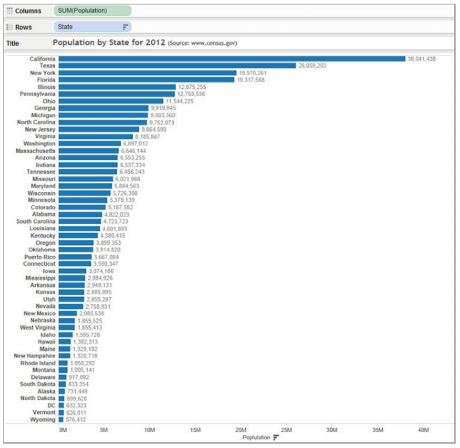United States population diagram

The dual axis chart requires the following items:

1. Running sum of state population
2. Percent of total population
3. The index table calculation function (to create the state ranking)
4. A Boolean calculation to derive the top 10 states (used in bar color)

The running sum of state population and percent of total population values will be created using a calculation. Below diagram shows the table calculation edit menu with the secondary switch activated.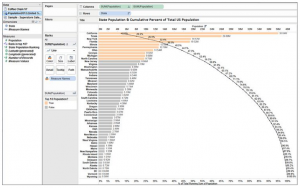Diagram of dual axis chart with population and the percent of total cumulative population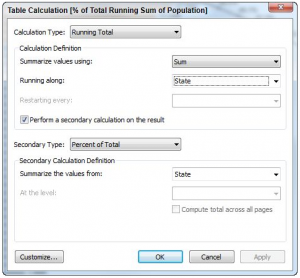Using a secondary table calculation diagram

The first running total calculation sums the state population along the scope of state. The bar chart is sorted by descending population values so the largest state is first in the table, followed by each lower ranked state in order. The secondary calculation uses the percent of total to derive the cumulative percent of total population that each state represents to the total United States population. Clicking  the OK button locks in the table calculation. The result is used to create the line chart with the tables displaying the cumulative total population at that row position.

### List Of Tableau Courses:Course Schedule
NameDates
Tableau TrainingJan 28 to Feb 12
Tableau TrainingJan 31 to Feb 15
Tableau TrainingFeb 04 to Feb 19
Tableau TrainingFeb 07 to Feb 22
Last updated: 27 January 2023